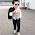We have discussed the classification of gear in our previous post, let us go ahead to understand the fundamental of terminology of gear used in industries. We will discuss the each term in respect of gear, but before understanding the terminology of gear we must focus over pressure angle of gear.

Normally there are three types of pressure angles are used in industries i.e. 14*1/20, 200 and 250.

A gear with higher pressure angle will have larger base and hence will be more capable to provide the higher torque. A gear with pressure angle of 250 will be more suitable to use in industries for better efficiency of gear and hence these days’ 250 pressure angle gears are used frequently.

Let us discuss the terminology of gear.

### Pitch Circle

Pitch circle is an imaginary circle that divides the gear teeth in two portion i.e. top lands and bottom lands or we can also say that pitch circle divides the gear teeth in addendum and dedendum.

When two gear meshes with each other, pitch circle of both gear will be tangent with each other and hence all calculation in respect of gear will be based on pitch circle. During meshing of two gears, pitch circle of both gears will meet at a point with each other and that point is known as pitch point and will also have similar linear velocity at that point.

### Pitch circle diameter

Diameter of pitch circle is called as pitch circle diameter, how you will measure the pitch circle diameter?
We can measure the diameter of pitch circle by measuring the distance between top of one gear tooth to bottom of opposite gear tooth.

### Pitch point

During meshing of two gears, pitch circle of both gears will meet at a point with each other and that point is known as pitch point.

### Top land

Top land is the top portion of gear tooth which will be extended above the pitch circle.

### Bottom land

Bottom land is the bottom portion of gear tooth which will be extended below the pitch circle.

Addendum is basically the radial distance between pitch circle and top land.

Addendum circle is basically an imaginary circle that passes through the addendum of gear teeth.

### Dedendum (b)

Dedendum is basically the radial distance between pitch circle and bottom land.

### Dedendum Circle

Dedendum circle is basically an imaginary circle that passes through the addendum of gear teeth.

### Base circle

Base circle is a circle from where involute portion of tooth profile will be produced.

### Circular Pitch

Distance which is measured along the pitch circle from a point on a gear tooth to the corresponding point on the adjacent gear tooth is called as circular pitch.
Circular pitch, PC= π. D/N = π m
Where, m is module (we will discuss module below)

### Circular thickness

Distance which is measured along the pitch circle from one side of gear tooth to opposite side of gear tooth i.e. the thickness of gear tooth will be called as circular thickness.

### Face width

Face width is called as the length of tooth in axial direction.

### Width of space

Width of space is basically the space, measured along pitch circle, between two teeth of a gear.

### Backlash

When two gear meshes with each other, the difference between the width of space between teeth and circular thickness of mating gear teeth is called as backlash.

### Module

Module is basically defined as the ratio of pitch circle diameter to the number of teeth
m= D/N

### Diametral pitch

Diametral Pitch is defined as the number of teeth per unit pitch circle diameter or the ratio of number of teeth to pitch circle diameter will be called as diametral pitch
Pd = N/D

### Outside diameter

Outside diameter is the major diameter in a gear.

### Root circle

Root circle is basically a circle that passes through the root of gear teeth and the diameter of root circle is called as root circle diameter.

### Whole depth

Whole depth is basically the sum of addendum and dedendum of gear teeth.
Ht= a +b

### Working depth

Working depth is basically the depth of engagement, when two mating gear meshes with each other. Working depth is expressed by HW.

### Clearance

Clearance is basically defined as the difference between the whole depth and working depth
C= Ht – HW

### Pressure Line

Pressure line is basically a common normal line at a point of contact of two mating gears and driving gear tooth will exert the force to the driven gear tooth along this common normal line.

### Pressure angle

Pressure angle is basically defined as the angle between pressure line and common tangent line at pitch circle of two mating gears. Sometimes we also say this angle as angle of obliquity.

Please provide your valuable feedback and comment.

1.2.